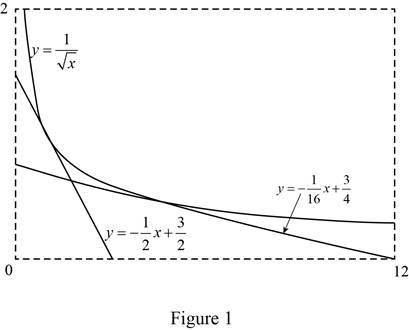# The slope of the tangent line to the curve at the given point.### Single Variable Calculus: Concepts...

4th Edition
James Stewart
Publisher: Cengage Learning
ISBN: 9781337687805### Single Variable Calculus: Concepts...

4th Edition
James Stewart
Publisher: Cengage Learning
ISBN: 9781337687805

#### Solutions

Chapter 2.6, Problem 10E

(a)

To determine

## To find: The slope of the tangent line to the curve at the given point.

Expert Solution

The slope of the tangent line to the curve y=1x at the point where x=a is m=12a32 or m=12a32, [a>0].

### Explanation of Solution

Given:

The equation of the curve is y=1x.

The curve passing through the points (1, 1) and (4,12).

Formula used:

The slope of the tangent curve y=f(x) at the point P(a,f(a)) is,

m=limxaf(x)f(a)xa (1)

Difference of square formula: (a2b2)=(a+b)(ab)

Calculation:

Obtain the slope of the tangent to the curve at the point x=a.

Since f(x)=1x, substitute f(a)=1a in equation (1),

m=limxaf(x)f(a)xa=limxa1x1axa=limxaaxaxxa=limxaax(xa)ax

Multiply both the numerator and the denominator by the conjugate of the numerator.

m=limxaax(xa)ax×a+xa+x

Apply the difference of squares formula,

m=limxa(a)2(x)2(xa)(a+x)ax=limxaax(xa)(a+x)ax=limxa(xa)(xa)(a+x)ax

Since the limit x approaches to a but not equal to a, cancel the common term xa(0) from both the numerator and the denominator,

m=limxa1(a+x)ax=1(a+a)a(a)=1(2a)a2

Perform the mathematical operations and compute the value of the function as shown below.

m=12a(a)=12(a)12(a)=12(a)32=12a32

Thus, the slope of the tangent line to the curve at the point x=a is m=12a32 or m=12a32, [a>0].

(b)

To determine

### To find: The equation of the tangent lines to the curve at the given points.

Expert Solution

The equation of the tangent lines to the curve y=1x at the points (1, 1) and (4,12) are y=12x+32 and y=116x+34 respectively.

### Explanation of Solution

Formula used:

The equation of the tangent line to the curve y=f(x) at the point is,

yf(a)=f(a)(xa) (2)

Calculation:

Obtain the equation of the tangent line at the point (1, 1).

Since the tangent line to the curve y=f(x) at (a,f(a)) is the line through the point (a,f(a)) whose slope is equal to the derivative of a, f(a)=12a32.

At the point (1, 1), consider a=1 and f(a)=1,

f(1)=12(1)32=12(1)=12

Substitute a=1, f(a)=1 and f(a)=12 in equation (2),

(yf(a))=f(a)(xa)y1=12(x1)y1=12x+12

Isolate y as shown below:

y=12x+12+1=12x+32

Thus, the equation of the tangent line is y=12x+32.

Obtain the equation of the tangent line at the point (4,12).

Since the tangent line to the curve y=f(x) at (a,f(a)) is the line through the point (a,f(a)) whose slope is equal to the derivative of a, f(a)=12a32.

At the point (4,12), take a=4 and f(a)=12

f(4)=12(4)32=12(4)4=12(4)(2)=116

Substitute a=2, f(a)=3 and f(a)=116 in equation (2).

(yf(a))=f(a)(xa)y12=116(x4)y12=116x+14

Isolate y as shown below.

y=116x+14+12=116x+1+24=116x+34

Thus, the equation of the tangent line is y=116x+34.

(c)

To determine

Expert Solution

### Explanation of Solution

Given:

The equation of the curve is y=1x.

The equation of the tangent lines are y=12x+32 and y=116x+34.

Graph:

Use the online graphing calculator to draw the graph of the functions as shown below in Figure 1.From Figure 1, it is noticed that the two lines y=12x+32 and y=116x+34 touches the curve y=1x at a unique point. That is, the two lines are tangent to the curve y=1x.

### Have a homework question?

Subscribe to bartleby learn! Ask subject matter experts 30 homework questions each month. Plus, you’ll have access to millions of step-by-step textbook answers!